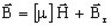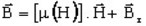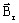# List of models for hard magnetic materials

## Models for hard magnetic materials

The various models available for the description of hard magnetic materials permanent magnets are presented in the following table.

Anisotropic material: linear approximationLinear magnet described by the Br module

Linear magnet described by the Br module, with Br linear function of the temperature

unidirectional

Linear magnet described by the Br Cartesian vector

Linear magnet described by the Br cylindrical vector

Linear magnet described by the Br spherical vector

vector
Anisotropic material: nonlinear approximationNonlinear magnet described by Hc and the Br module

Nonlinear magnet described by Hc and the Br module, plus knee adjustment

Nonlinear magnet described by a spline

unidirectional

Specific models are also provided (see table below). Their mode of use is presented in § Spatial model / user model.

Magnetic property …
… spatial linear magnet ( μr anddescribed via spatial formula )

## Direction of magnetization (orientation)

Magnetization is a vector quantity which can be defined:

• either by a module and a direction (in a coordinate system)
• or by components in a coordinate system

These two modes of definition provided in Flux are presented in the two tables below.

In a model of the “unidirectional” type

the mathematical model and the direction of magnetization are dissociated.

Definition Use
Module of Br

Direction (in a plane XOY)

• unidirectional